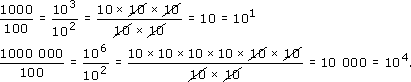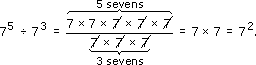Squares, roots and powers

This free course is available to start right now. Review the full course description and key learning outcomes and create an account and enrol if you want a free statement of participation.

Free course

2.4 Dividing powers

Now consider division in the same way. For example:So 103 ÷ 102 = 101 and 106 ÷ 102 = 104, which suggests that dividing the powers of ten means subtracting the powers. You can see that the two tens underneath have cancelled with two of the tens on top in each case, which shows why the number of tens underneath is subtracted from the number on top. This argument applies equally well to any number. For example:Work out 75 ÷ 73 on your calculator to check this.

To divide two powers of the same number, subtract the powers.

MU120_4M4